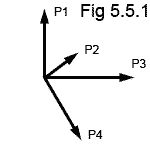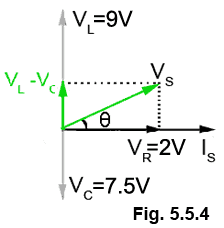# Phasor Quiz

Try this quiz, based on the information you can find in Module 5. Hopefully it won´t be too difficult. Submit your answers to see how well you did. If you got any answers wrong, you´ll be told where to find the right answer, so you can learn more about AC circuits and Phasors before you have another try.

### 1.

In an inductor, an increase in the value of the internal resistance has the effect of: (Complete the sentence).

### 2.

In a capacitor: (Complete the sentence).

### 3.

Which property of a sine wave does the length of a phasor represent?

### 4.Refer to Fig. 5.5.1: Which of the following statements about Fig 5.5.1 is true?

### 5.Refer to Fig 5.5.2: What is the phase difference θ between the supply voltage and the supply current?

### 6.Refer to Fig 5.5.3: Which of the phasor diagrams describes a CR series Circuit?

### 7.For the phasor diagram shown in Fig 5.5.4, calculate the phase angle θ between IS and VS.

### 8.

In a parallel LCR circuit, which of the following properties is used as the reference phasor?

### 9.

A phasor representing a 1kHz sine wave will rotate through how many complete radians in one second?

### 10.

In a phasor diagram, the frequency of each of the waves shown by the phasors is:

Top of Page.>